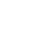# scipy.io（输入和输出）包用于读写各种格式的文件

scipy.io(输入和输出)包用于读写各种格式文件。scipy.io支持的格式很多，下面列出了几种常用格式：

MATLAB

Matlab 格式是最常用的。

``````import scipy.io as sio
import numpy as np
# 保存mat文件
vect = np.arange(20)
sio.savemat('array.mat', {'vect':vect})
# 加载文件
print (mat_file_content)``````

``````{'__header__': b'MATLAB 5.0 MAT-file Platform: posix, Created on: Sun Jun 30 10:51:57 2019', '__version__': '1.0', '__globals__': [], 'vect': array([[ 0,  1,  2,  3,  4,  5,  6,  7,  8,  9, 10, 11, 12, 13, 14, 15,
16, 17, 18, 19]])}``````

``````import scipy.io as sio
mat_file_content = sio.whosmat('array.mat')
print (mat_file_content)``````

``[('vect', (1, 20), 'int64')]``

《scipy.io（输入和输出）包用于读写各种格式的文件》